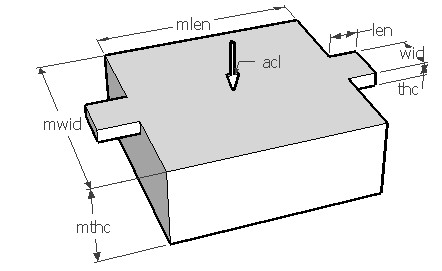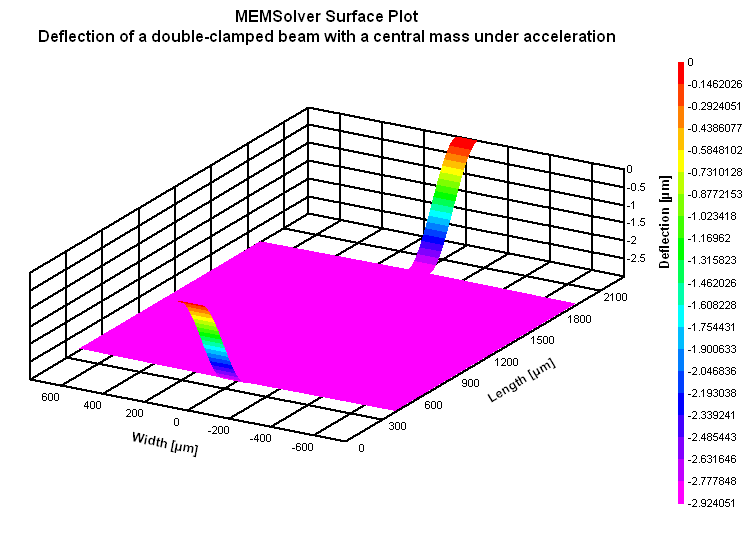MACLAMP,len,wid,thc,mlen,mwid,mthc,acl,den,ymod,sel
Bending of a double-clamped beam with a central mass under acceleration

len          length of beam in µm
wid         width of beam in µm
thc          thickness of beam in µm
mlen       length of the mass in µm
mwid      width of the mass in µm
mthc       thickness of the mass in µm
acl          acceleration as multiples of g
an input value of 10 means 98.5m/s2
den         density of the material in kg/m3
ymod      Young's modulus of the material in GPa
sel          number denoting the selected result.
Use 1 for maximum stress, 2 for deflection and 3 for spring constantNotes

An inertial mass suspended from two or more clamped beams is a structure often used in MEMS accelerometers and other motion sensitive devices. A typical structure is as shown above. When an acceleration load is applied in the direction shown, the mass will undergo a displacement till the spring action of the beams balance out the acceleration load.

Use this form to calculate the displacement of the mass due to the acceleration load and the resulting stress in the beams.  If there are more springs supporting the mass connected in parallel, the effective spring constant will be the sum of spring constants of all the beams.

The X-Y plot gives the distribution of stress on the beam surface. It shows that the stress changes from positive maximum to negative maximum along the length of the beam. When the mass is pushed down due to inertia, the surface stress near the clamped edge of the beam will be tensile and that near the mass will be compressive. The 2D and 3D surface plots show the deflection of the beam mass system under acceleration.Assumptions

-The default material is Silicon with a Young's modulus of 180GPa.
-The beam has uniform cross section.
-The weight of the beam and mass is uniformly distributed. Deflection due to weight of the mass is negligible.
-There is no other load acting on the structure other than acceleration load perpendicular to the mass.
-The bending of the mass is negligible.
-Damping is not considered.